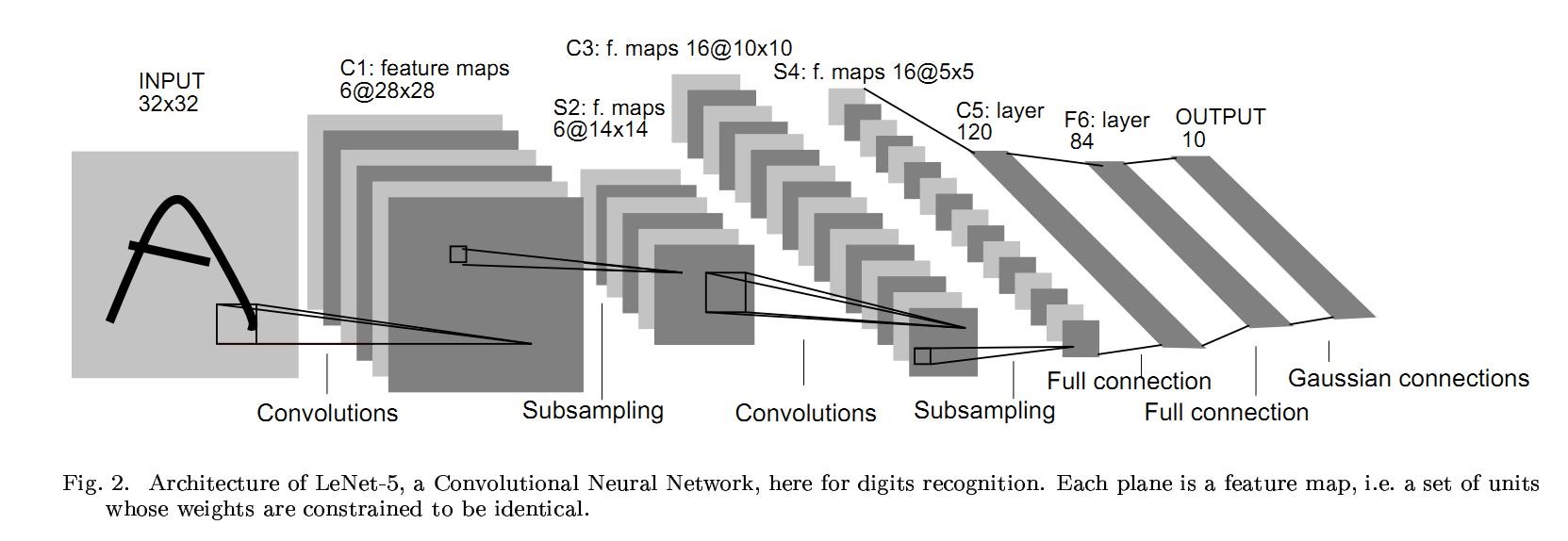zoukankan      html  css  js  c++  java

# 0601-利用pytorch的nn工具箱实现LeNet网络

pytorch完整教程目录：https://www.cnblogs.com/nickchen121/p/14662511.html

# 一、引言

首先再次安利一篇文章，这篇文章详细介绍了如果使用一个深度神经网络去实现人脸识别，这里面对卷积、池化、全连接、激活函数都有一个较为详细的解释，看完这篇文章，再来看这篇文章，相信会有一种醍醐灌顶之效：06-01 DeepLearning-图像识别

因此在 torch 中，`torch.nn` 的出现就是专门为神经网络设计的模块化接口，nn 构建与 autograd 之上，可以用来定义和运行神经网络。其中 `nn.Module` 是 nn 中最重要的类，可以把它看作是一个网络的封装，包含网络中各层的定义和 forward 方法，调用 `forward(input)` 方法，可以轻松的实现前向传播。

接下来我们将以卷积神经网络 LeNet 网络为例，看看如何用 `nn.Module` 实现，其中 LeNet 网络架构如下图所示：上述图示是一个基础的前向传播网络：接收输入，经过层层传递运算，得到一个输出。

当然，这篇文章的重心是告诉我们如何利用 nn 这个工具箱搭建一个基础的神经网络架构，至于 nn 的具体用法将在未来的分享中详细介绍，也就是说这一篇文章只是笼统的介绍 nn，只要看清楚本篇文章的大体脉络即可，至于细节未来都会一一介绍。

# 二、定义网络

定义网络的时候需要继承 `nn.Module`，并实现它的的 forward 方法，把网络中具有可学习参数的层放到构造函数 `__init__` 中。如果某一层不具有可学习的参数，则即可以放在构造函数中，也可以不放入。

``````import torch as t
import torch.nn as nn
import torch.nn.functional as F
from torch.autograd import Variable as V

class Net(nn.Module):
def __init__(self):
super(Net, self).__init__()  # nn.Module 子类的函数必须在构造函数中执行父类的构造函数

# 卷积层
self.conv1 = nn.Conv2d(1, 6,
5)  # '1'表示输入图片为单通道，‘6’表示输出通道数，‘5’表示卷积核为 5*5
self.conv2 = nn.Conv2d(6, 16, 5)

# 仿射层/全连接层，y = Wx + b
self.fc1 = nn.Linear(16 * 5 * 5, 120)
self.fc2 = nn.Linear(120, 84)
self.fc3 = nn.Linear(84, 10)

def forward(self, x):
# 卷积-》激活-》池化
x = F.max_pool2d(F.relu(self.conv1(x)), (2, 2))
x = F.max_pool2d(F.relu(self.conv2(x)), 2)

# reshape，‘-1’表示自适应
x = x.view(x.size(), -1)
x = F.relu(self.fc1(x))
x = F.relu(self.fc2(x))
x = self.fc3(x)

return x

net = Net()
net
``````
``````Net(
(conv1): Conv2d(1, 6, kernel_size=(5, 5), stride=(1, 1))
(conv2): Conv2d(6, 16, kernel_size=(5, 5), stride=(1, 1))
(fc1): Linear(in_features=400, out_features=120, bias=True)
(fc2): Linear(in_features=120, out_features=84, bias=True)
(fc3): Linear(in_features=84, out_features=10, bias=True)
)
``````

通过 `nn.module` 成功定义网络结构后，有3个点需要注意：

1. 只要在 `nn.Module` 的子类中定义了 forward 函数，backward 函数就会自动实现
2. 网络的可学习参数通过 `net.parameters()` 返回，`net.named_parameters` 可同时返回可学习的参数和名称
3. 只有 Variable 才有自动求导功能，因此forward 函数的输入和输出都是 Variable，所以在输入时，需要把 Tensor 封装成 Variable
``````params = list(net.parameters())
len(params)
``````
``````10
``````
``````for name, parameters in net.named_parameters():
print(f'{name}: {parameters.size()}')
``````
``````conv1.weight: torch.Size([6, 1, 5, 5])
conv1.bias: torch.Size()
conv2.weight: torch.Size([16, 6, 5, 5])
conv2.bias: torch.Size()
fc1.weight: torch.Size([120, 400])
fc1.bias: torch.Size()
fc2.weight: torch.Size([84, 120])
fc2.bias: torch.Size()
fc3.weight: torch.Size([10, 84])
fc3.bias: torch.Size()
``````
``````input = V(t.randn(1, 1, 32, 32))  # 定义输入
out = net(input)
out.size()  # 输出的形状
``````
``````torch.Size([1, 10])
``````
``````net.zero_grad()  # 所有参数的梯度清零
out.backward(V(t.ones(1, 10)))  # 反向传播
``````

注：`torch.nn`只支持 mini-batches，不支持一次输入一个样本。如果想一次输入一个样本，可以用 ` `input.unsqueeze(0)` 把 batch_size 设置为 1。例如，`nn.Conv2d`输入必须是 4 维的，形如`nSamples × nChannels × Height × Width`，可以让 nSample 设为 1，也就是 `1 × nChannels × Height × Width`

# 三、损失函数

nn 实现了神经网络中大多数的损失函数，例如 `nn.MSELoss` 计算均方误差，`nn.CrossEntropyLoss` 计算交叉熵损失。

``````output = net(input)  # net(input)的输出的形状是(1,10)
target = V(t.arange(0, 10)).view(1, 10).float()
criterion = nn.MSELoss()
loss = criterion(output, target)
loss
``````
``````tensor(28.5546, grad_fn=<MseLossBackward>)
``````

``````input -> conv2d -> relu -> maxpool2d -> conv2d -> relu -> maxpool2d
-> view -> linear -> relu -> linear -> relu -> linear
-> MSELoss
-> loss
``````

当调用 `loss.backward()` 时，该图会动态生成并自动微分，也会自动计算图中参数的导数

``````# 运行.backward，观察调用之前和调用之后的 grad
loss.backward()
``````
``````反向传播之前conv1.bias 的梯度：tensor([0., 0., 0., 0., 0., 0.])
反向传播之后conv1.bias 的梯度：tensor([ 0.1055,  0.0943, -0.1617,  0.0416, -0.0787,  0.0285])
``````

# 四、优化器

在反向传播完成所有参数的梯度计算后，还需要使用优化方法更新网络的权重和参数。在 `torch.optim` 中实现了深度学习中绝大多数的优化方法，这里不详解介绍，未来会详细介绍，目前能成为一个合格的调包侠即可。

``````import torch.optim as optim

# 新建一个优化器，指定要调整的参数和学习率
optimizer = optim.SGD(net.parameters(), lr=0.01)

# 计算损失
output = net(input)
loss = criterion(output, target)

# 反向传播
loss.backward()

# 更新参数
optimizer.step()
``````

# 五、数据加载和预处理

在深度学习中数据加载和预处理是非常麻烦的，但是 torch 提供了一些列简化和加快处理数据的工具，未来我们也都会详解介绍。并且torch 也把一些常用的数据集都保存在了 torchvision 中。

# 六、Hub模块简介

上面给出了定义一个完整的神经网络的流程，但是还是太复杂了，如果你仅仅只是想使用一个神经网络模型完成自己的一个小demo，而不是自己费尽心思的写一个模型出来，那么hub模块就可以满足你的要求，你可以从hub模块官网获取任何一个已存的模块，然后丢入你的数据就可以获得结果，而不需要自己巴拉巴拉写一堆代码。也就是说，别人用轮子造航母，你直接把航母拿来用。

以下就是hub模块的大概用法，当然，更详细的内容可以去官网查询：

``````import torch

model = torch.hub.load('pytorch/vision:v0.4.2', 'deeplabv3_resnet101', pretrained=True)  # 加载模型，第一次加载需要一点点时间
model.eval()  # 释放模型
``````

# 七、总结

上面笼统的介绍了如何利用 nn 这个工具箱去搭建一个神经网络，但是只给出了一个流程，很多细节我们还没有详细解释，但这已经足够了。

下一篇文章我们就将详细介绍 nn 工具箱的各种细节方面的东西，等你看完下一篇文章如果再跳回来看这篇文章，相信定会有醍醐灌顶之效。

• 相关阅读:
Python3---常见函数---super()
Python3---常见函数---type()
Python3---面对对象
Python3---BeautifulSoup---节点选择器
Python3---Beautiful Soup
0X01应用程序黑客技术
Python3---标准库---re
(trie) UVA
（trie）UVALive
（平方分割）POJ 2104 K-th Number
• 原文地址：https://www.cnblogs.com/nickchen121/p/14688319.html